College Physics : Spring Force

Example Questions

Example Question #1 : Spring Force

A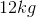bowling ball is dropped from a height of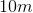onto a spring. If the spring is to compressed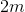before halting the ball, what is the spring constantof the spring?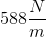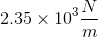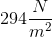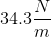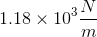Explanation:

This questions requires an understanding of the conversion of gravitational potential energy to potential energy stored in the compression of a spring. The ball's initial potential energy is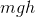whereis the mass of the ball,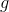is the acceleration due to gravity, andis the height of the ball. The energy stored in a compressed spring is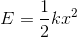where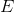is the potential energy,is the spring constant (typically given in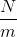) andis the compression of the spring. By setting the gravitational potential energy equal to the energy stored in the spring, we can solve for the spring constant: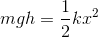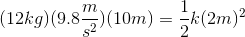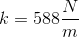Example Question #1 : Spring Force

A block with mass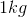is attached to a vertically hanging spring with negligible mass and spring constant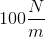and is allowed to reach a new equilibrium position. Approximately how far does the spring stretch from its initial equilibrium position?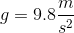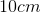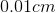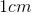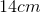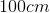Explanation:

The new equilibrium position will be where the spring force is opposite in direction and equal in magnitude to the gravitational force on the mass. This occurs where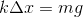where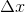is the displacement of the spring from equilibrium. Solving for, we get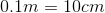.

Example Question #2 : Spring Force

Supposed that an object attached to a spring with a spring constant of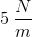is displaced by a certain amount. The elastic potential energy contained within the spring is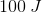. What is the magnitude of the force acting on the object from the spring at this instant?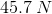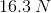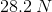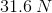Explanation:

The first step in answering this question is to recognize that we're dealing with two components of a spring; force and energy. Hence, we'll need to utilize both equations. Because both of them share the term for the displacement of the object, that will be the variable that links the two expressions.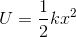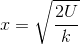Having isolated the variable for displacement, we can plug this value into the force equation.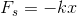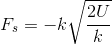Now, we can plug in the values given to us in the question stem.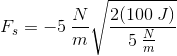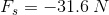Note that the negative sign shown here indicates the direction of the force. This is because the force is acting in the direction opposite the displacement of the object. Since the question stem asks for the magnitude of force, we don't need to include the negative sign in our answer.

All College Physics Resources